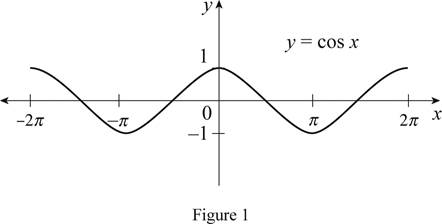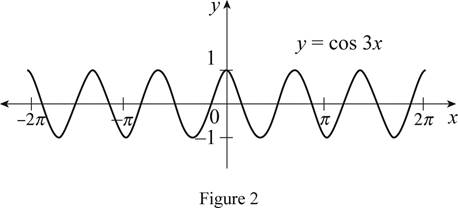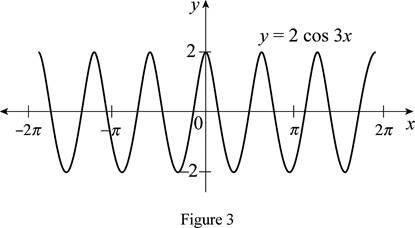# The function y = cos x by hand without plotting any points and then graph the function y = 2 cos 3 x .### Single Variable Calculus: Concepts...

4th Edition
James Stewart
Publisher: Cengage Learning
ISBN: 9781337687805### Single Variable Calculus: Concepts...

4th Edition
James Stewart
Publisher: Cengage Learning
ISBN: 9781337687805

#### Solutions

Chapter 1.3, Problem 13E
To determine

## To sketch: The function y=cosx by hand without plotting any points and then graph the function y=2cos3x .

Expert Solution

### Explanation of Solution

The standard graph of the function y=cosx is roughly drawn and shown below in Figure 1.Then, draw the graph of y=cos3x , by shrink the graph y=cosx horizontally by a factor of 3. Thus, the graph of y=cos3x is shown below in Figure 2.Observe that Figure 2 is compressed from Figure 1 by a factor of 3.

To draw the graph of y=2cos3x , stretch the graph y=cos3x vertically by a factor of 2. Thus, the graph of y=2cos3x is shown below in Figure 3.Observe that Figure 3is obtainedfrom Figure 1 in such a way that it is shrunkhorizontally by a factor of 3 and then stretched vertically by a factor of 2.

### Have a homework question?

Subscribe to bartleby learn! Ask subject matter experts 30 homework questions each month. Plus, you’ll have access to millions of step-by-step textbook answers!